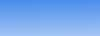产品展示

 主要产品系列Cortex ARM7 80C51

# 深入理解指针函数和函数指针

## 1.指针函数的定义

“(函数参数表列)”中的括号为函数调用运算符，在调用语句中，即使函数不带参数，其参数表的一对括号也不能省略。其示例如下：

int *pfun(int, int);

int *(pfun(int, int));

int (*pfun)(int, int);

## 2. 用函数指针作为函数的返回值

int (*ff(int))(int *, int);

int (*(ff(int)))(int *, int); // 用括号将ff(int)再括起来

int (*PF)(int *, int);

typedef int (*PF)(int *, int);

PF ff(int);

1 #include<stdio.h>
2 #include <assert.h>
3 double GetMin(double *dbData, int iSize) // 求最小值
4 {
5 double dbMin;
6 int i;
7
8 assert(iSize>0);
9 dbMin=dbData;
10 for (i=1; i<iSize; i++){
11 if (dbMin>dbData[i]) {
12 dbMin=dbData[i];
13 }
14 }
15 return dbMin;
16 }
17
18 double GetMax(double *dbData, int iSize) // 求最大值
19 {
20 double dbMax;
21 int i;
22
23 assert(iSize>0);
24 dbMax=dbData;
25 for (i=1; i<iSize; i++){
26 if (dbMax< dbData[i]) {
27 dbMax=dbData[i];
28 }
29 }
30 return dbMax;
31 }
32
33 double GetAverage(double *dbData, int iSize) // 求平均值
34 {
35 double dbSum=0;
36 int i;
37
38 assert(iSize>0);
39 for (i=0; i<iSize; i++)
40 {
41 dbSum+=dbData[i];
42 }
43 return dbSum/iSize;
44 }
45
46 double UnKnown(double *dbData, int iSize) // 未知算法
47 {
48 return 0;
49 }
50
51 typedef double (*PF)(double *dbData, int iSize); // 定义函数指针类型
52 PF GetOperation(char c) // 根据字符得到操作类型，返回函数指针
53 {
54 switch (c)
55 {
56 case 'd':
57 return GetMax;
58 case 'x':
59 return GetMin;
60 case 'p':
61 return GetAverage;
62 default:
63 return UnKnown;
64 }
65 }
66
67 int main(void)
68 {
69 double dbData[]={3.1415926, 1.4142, -0.5,999, -313, 365};
70 int iSize=sizeof(dbData)/sizeof(dbData);
71 char c;
72
73 printf("Please input the Operation :\n");
74 c=getchar();
75 printf("result is %lf\n", GetOperation(c)(dbData,iSize)); // 通过函数指针调用函数
76 }管理员 | 首页 | 产品 |  联系我们 © 2006 WeBoch.Com.cn 版权所有：深圳市伟博创科技有限公司 地址：深圳市福田区振华路苏发大厦305栋405 电话：0755-83240703 传真：0755-83240724